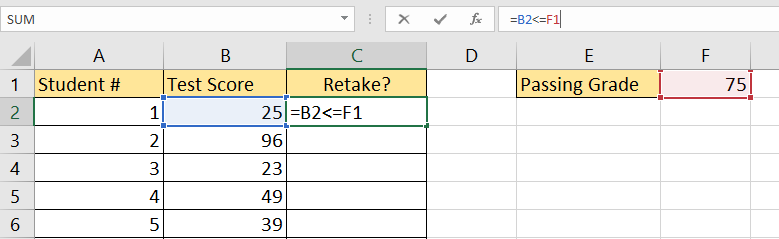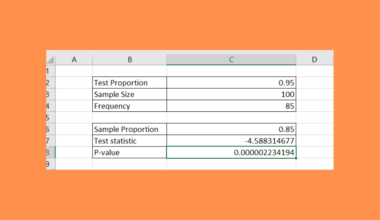# How to Use Less Than or Equal to Operator in Excel

The less than or equal to operator in Excel is useful when you need to know if a number is of equal or lesser value than another number.

The less than or equal to operator can also be used to compare strings.

When can we use this operator?

Suppose you have a list of test scores for a 100 item test. You want to know how many students failed the exam, which happens when they score equal to or lower than 75. If they get a score equal to or lower than 75, they’ll be eligible for a retake of the exam. How do we create a column to determine which students need to retake the exam?

With the “>=” operator, it becomes quite easy to compare any value to the passing grade, which is 75.

This use case is just one way we can use comparison operators. We can use the “>” operator to return values strictly less than 75. We can also use the greater than operator to find out which numbers are greater than 75.

These operators work for all types of numbers. Both values can also be cell references, provided that the values contained in both are valid numbers.

Now that we know what these operators can do, let’s look at how we can use them on an actual spreadsheet.

## A Real Example of Using the Less Than or Equal to Operator in Excel

Let’s take a look at a real example of a spreadsheet that uses the less than or equal to operator.

In the table below, we have a list of test scores for a 100-point exam. Column C uses a comparison operator to determine whether the student will have to retake the exam or not. Cell F3 also contains another formula that computes how many students will have to retake the exam given the passing grade and the range of scores.To get the values in Column C, we just need to use the following formula:

`=B2<=\$F\$1`

This formula simply compares the row’s corresponding test score with the fixed value in the cell that holds the passing grade.

To get the value in cell F3, we used the following formula:

`=COUNTIF(B:B;"<=75")`

The COUNTIF function accepts two arguments: the cell range to count and the condition that defines which cells are to be counted. In this case, we’ve specified the function to count Column B and only to count cells if the value is less than or equal to 75.

If you’re ready to try out the less than or equal to operator in Excel, let’s begin writing it ourselves!

## How to Use Less Than or Equal to Operator in Excel

This section will guide you through each step needed to start using comparison operators in Microsoft Excel. You’ll learn how we were able to use the less than or equal to operator to determine failing grades in a spreadsheet.

Follow these steps to start using the less than or equal to operator in Excel:

1. First, select the cell that will hold the first instance of our comparing formula. In this example, we’ll start with cell C2.2. Next, we must write down our comparison formula. In this example, we will first compare the test score in cell B2 with the passing grade in cell F1. We will use the “<=” operator to indicate that we only want students who scored lower than or equal to 75 to retake the exam.3. Before we fill out the rest of Column C, it would be helpful to convert the cell reference to F1 into an absolute reference. An absolute reference for that cell would look like this: \$F\$1.4. Fill out the entire row by dragging down the formula we wrote in cell C2. We now know which students will have to retake the exam.Besides outputting Boolean values, we can also use comparison operators to control the output of other formulas such as `COUNTIF`. In the example below, we will show how to use the “<=” operators with a COUNTIF function.

1. First, write down the formula for COUNTIF. Indicate the two arguments needed: the column to count, and the criteria to check. Below, we assigned “<=75” to be our criteria.2. Hit the Enter key to return the final count. In this example, we counted 32 students who will be eligible to retake the exam due to their low scores.

1. Do the comparison operators work with date values?
Yes. Excel considers valid date values to be integers formatted in a specific way. For example, the formula =DATE(2021,01,01)<=DATE(2022,01,01) will return TRUE since January 1st 2021 comes before January 1st 2022.

This guide should be all you need to start using the comparison operators, such as the less than or equal to operator in Excel. This step-by-step tutorial shows how simple it is to use comparison operators with other functions such as `IF` or `COUNTIF` to give your formula a conditional element.

The less than or equal to operator is just one way to analyze the data in your Excel spreadsheets. With so many other Excel functions out there, you can surely find one that suits your use case.

Are you interested in learning more about what you can do in Microsoft Excel? Make sure to subscribe to our newsletter to be the first to know about the latest guides and tutorials from us.### Get emails from us about Excel.

Our goal this year is to create lots of rich, bite-sized tutorials for Excel users like you. If you liked this one, you'd love what we are working on! Readers receive ✨ early access ✨ to new content.

##### You May Also Like## How to Add a Stacked Fraction in Excel

This tutorial will explain how you can add a stacked fraction in Excel. Excel supports fractions as a…## How to Convert Decimals to Fractions in Excel

This guide will explain how you can convert decimals to fractions in Excel. Excel includes functions and custom…## How to Perform a One-Proportion Z-Test in Excel

This guide will explain how to perform a one-proportion Z-test in Excel. We can use the one proportion…## Make Multiple Selections from Drop-down List in Excel

This guide will explain how to allow multiple selections in a drop-down list in Excel. We’ll use Excel…